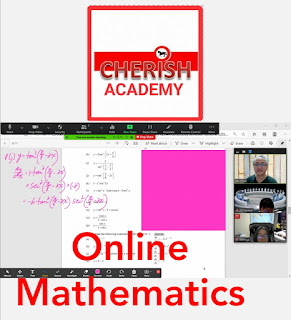## Tuesday, October 1, 2013

### Quick March Revision on Quadratic Equation 2016

☎️+6566189386 for steps by steps solutions .
We provide small group coaching and 121 coaching through Skype and Whatsapp

Maths Quick Revision for sec 2/3/4 students
Master My Maths
1. Factorize
a) x ² -25
b) 2x ² - 18
c) 25x ² -4 x ² y ²
d) 16 x ⁴ -16
e) 2 m ² - 4m
f) 2x ² - 2x -12
g)x ² -5x -6
h) 2(x ² -4) - x-2
i) x ² /9 - 16
2 . Factorise the followings :
a) (x-y) (2p + 3q ) -(y-x) ( 4p + 5q)
b) 4a ²  x + 12 a ²  y - 3y - x
c) 4a ²  - 9 ( a+b) ²
d) 2x ² -14x +24
e) x ²  - 4xy + 4y ²  -1
f) m ⁵ - m
g) mp -q + mq -p
h) 8-2( x-2) ²  and hence draw the graph stating the x and y intercepts and the line of symmetry.
3a) Given 9x ²   -12xy + 4y ²   =0 , find the value of 2x/y
b)Given that ax-2ay-3bx+4by =0, find 3x/4y in terms of a and b
4) solve the following
a) x ² -9 = 0
b) 2(2x -1) ² - 8 = 0
c) x(x +2) = 0
d) 3x ² - 6 = 0
e) a ² - a = 6
f) x/2-x/4 -1 = 0
g) (x -3)(x+2) =0
h) x ² +2 = 2
I) x ² - 2x + 1 = 0
5)Solve using perfect square method
a) 2 x ² -6x + 15 =0
b) x ² = 4x + 3
6 ) solve using formulae
a) -x ² - 4 x -2=0
b) 3 a ² -12 a = 9